Vision C2 Alternating Vision & Reality

Android Applications

3. Orbital Speed Calculator Source Code (App Inventor)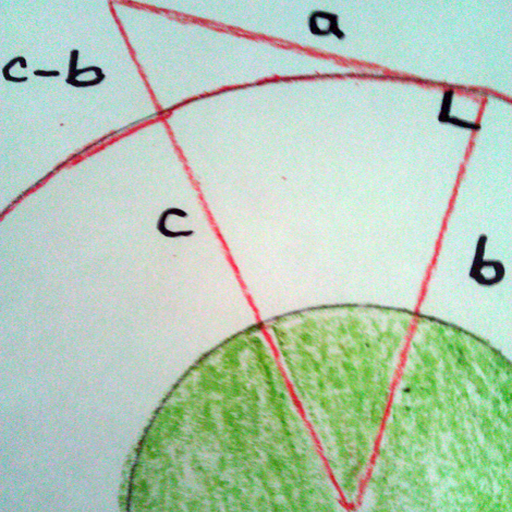You input a known orbit into this program. It will then calculate the mass of the body being orbited. You then input a new altitude in terms of radius and it will calculate the speed required to be in orbit at that distance.
Screen Shots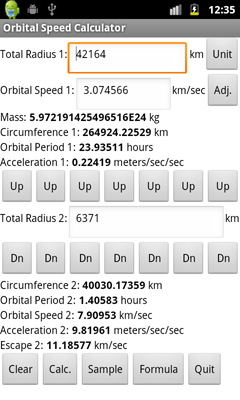Main Menu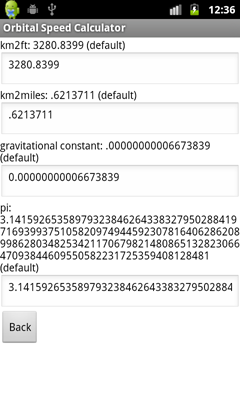Adjust Menu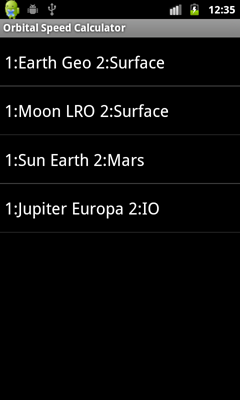Sample Menu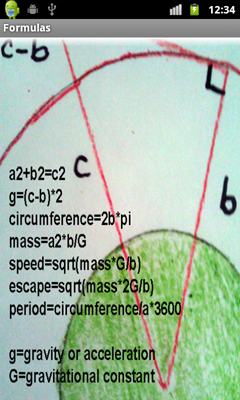Formulas Screen
Instructions

This program calculates an orbital speed based upon a known orbit. Upon startup, this program uses the altitude for a Geostationary orbit which is 42164 kilometers and speed of 3.074566 km/sec. The given value from Wikipedia is 3.0746 km/sec. The default secondary altitude is 6371 km which is the average radius from the center of the Earth at the surface. This program uses the Pythagoras theorem to calculate the gravity at the surface of the Earth as 32.21656 feet per second per second.

Details
• a is the distance object 1 travels in one second. c-b is the distance object 1 falls in one second.
• The Total Radius 1 and Orbital Speed 1 must be very precise to compute mass as the same as found on the internet.
• Assuming a Geostationary orbit is exactly 42164 km with a speed of 3.074566 km/sec, I adjusted the gravitational constant to .00000000006673839 instead of .00000000006673840 to compute the mass of the Earth as 5.97219E24kg. 5.97219E24kg is the value I found on Wikipedia.
• Starting with Earth orbit circumference of 940,000,000 km, you can input 149605646.5 as Total Radius 1, 29.783945 as Orbital Speed 1 to come up with 1.9885508E30 as the mass of the Sun. If the nice round number of 30 km/sec is more correct then the circumference is wrong.
• Assuming an exact LRO radius of 1788 (50 km above the Moon) and an LRO speed of 1.6560712523 we get the known mass of the Moon.
• I used a Total Radius 2 for Mars as 228924066 to come up with the known orbital speed of 24.077 km/sec
• Notice that the acceleration or gravity on the surface of the moon of 5.32612 ft/sec/sec is almost exactly 1/6th that of the Earth (32.21656 ft/sec/sec)
Hints

• Since distance equals 1/2 gravity times time squared, an object falling exactly 16 feet in one second equals a gravity or acceleration of 32 feet per second per second. Also see "Calculus for Dummies" page 86 to see how an object falling 16 feet in one second computes to an acceleration of 32 ft/sec/sec.
• The program uses the formulas: a squared plus b squared equals c squared, acceleration equals (c minus b) times 2, velocity equals the square root of mass times the gravitational constant over radius and to compute mass we use - a squared times b over the gravitational constant.
• Quirks

• You can input a Radius less than the surface of the Earth or other body, but the data will be incorrect, obviously because there is mass above the orbiting object which this program does not calculate.

A web site of Scott Teee Murphy